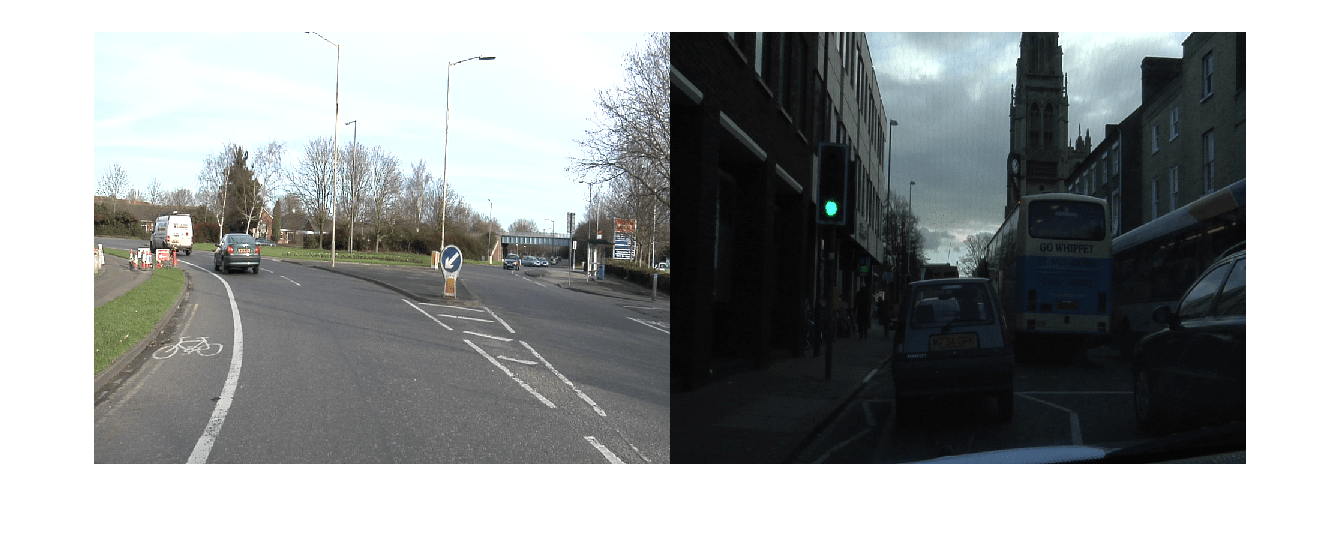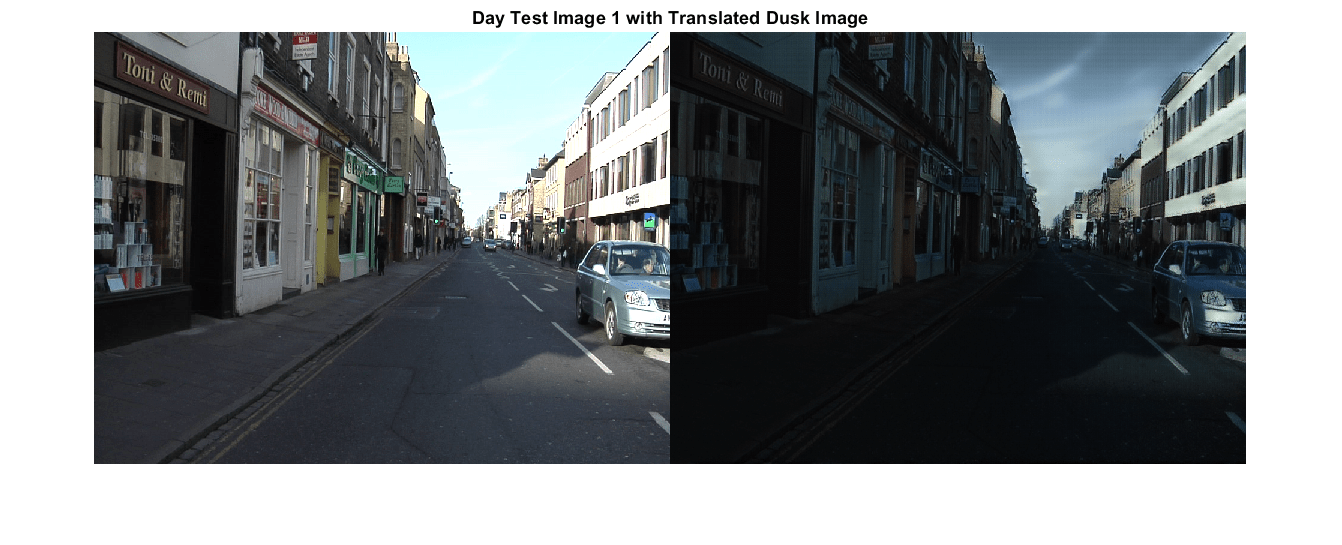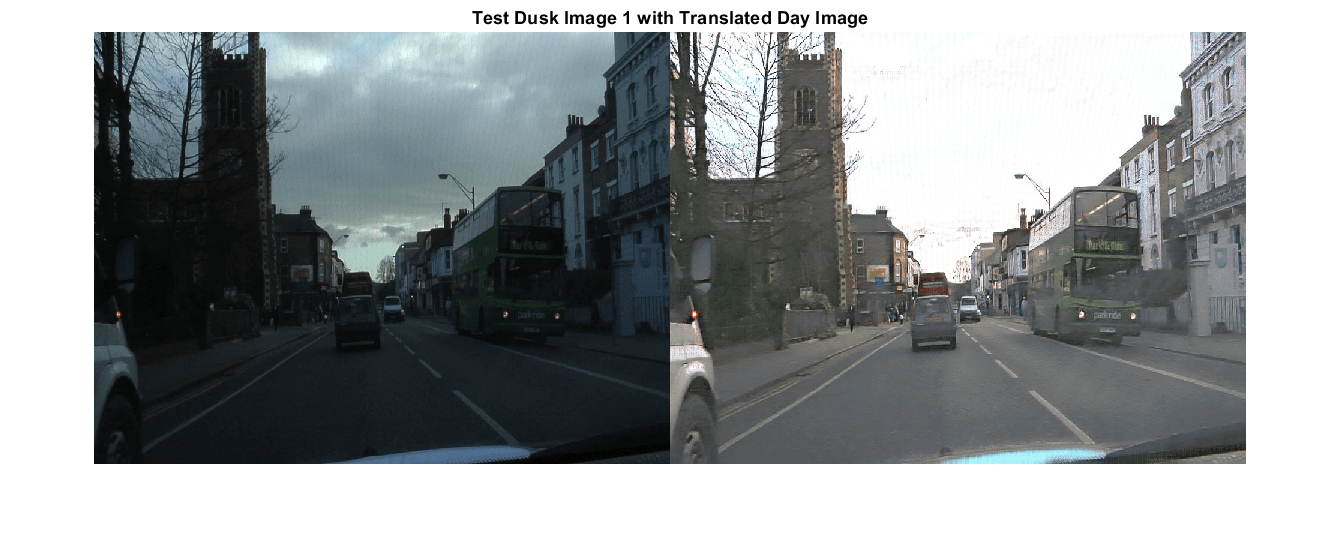# Unsupervised Day-to-Dusk Image Translation Using UNIT

This example shows how to translate images between daytime and dusk lighting conditions using an unsupervised image-to-image translation network (UNIT).

Domain translation is the task of transferring styles and characteristics from one image domain to another. This technique can be extended to other image-to-image learning operations, such as image enhancement, image colorization, defect generation, and medical image analysis.

UNIT  is a type of generative adversarial network (GAN) that consists of one generator network and two discriminator networks that you train simultaneously to maximize the overall performance. For more information about UNIT, see Get Started with GANs for Image-to-Image Translation.

This example uses the CamVid data set  from the University of Cambridge for training. This data set is a collection of 701 images containing street-level views obtained while driving.

Specify `dataDir` as the desired location of the data. Download the CamVid data set using the helper function `downloadCamVidImageData`. This function is attached to the example as a supporting file.

```dataDir = fullfile(tempdir,"CamVid"); downloadCamVidImageData(dataDir); imgDir = fullfile(dataDir,"images","701_StillsRaw_full");```

### Load Day and Dusk Data

The CamVid image data set includes 497 images acquired in daytime and 124 images acquired at dusk. The performance of the trained UNIT network is limited because the number of CamVid training images is relatively small, which limits the performance of the trained network. Further, some images belong to an image sequence and therefore are correlated with other images in the data set. To minimize the impact of these limitations, this example manually partitions the data into training and test data sets in a way that maximizes the variability of the training data.

Get the file names of the day and dusk images for training and testing by loading the file `camvidDayDuskDatasetFileNames.mat`. The training data sets consist of 263 day images and 107 dusk images. The test data sets consist of 234 day images and 17 dusk images.

`load("camvidDayDuskDatasetFileNames.mat");`

Create `imageDatastore` objects that manage the day and dusk images for training and testing.

```imdsDayTrain = imageDatastore(fullfile(imgDir,trainDayNames)); imdsDuskTrain = imageDatastore(fullfile(imgDir,trainDuskNames)); imdsDayTest = imageDatastore(fullfile(imgDir,testDayNames)); imdsDuskTest = imageDatastore(fullfile(imgDir,testDuskNames));```

Preview a training image from the day and dusk training data sets.

```day = preview(imdsDayTrain); dusk = preview(imdsDuskTrain); montage({day,dusk})```### Preprocess and Augment Training Data

Specify the image input size for the source and target images.

`inputSize = [256,256,3];`

Augment and preprocess the training data by using the `transform` function with custom preprocessing operations specified by the helper function `augmentDataForDayToDusk`. This function is attached to the example as a supporting file.

The `augmentDataForDayToDusk` function performs these operations:

1. Resize the image to the specified input size using bicubic interpolation.

2. Randomly flip the image in the horizontal direction.

3. Scale the image to the range [-1, 1]. This range matches the range of the final `tanhLayer` (Deep Learning Toolbox) used in the generator.

```imdsDayTrain = transform(imdsDayTrain, @(x)augmentDataForDayToDusk(x,inputSize)); imdsDuskTrain = transform(imdsDuskTrain, @(x)augmentDataForDayToDusk(x,inputSize));```

### Create Generator Network

Create a UNIT generator network using the `unitGenerator` function. The source and target encoder sections of the generator each consist of two downsampling blocks and five residual blocks. The encoder sections share two of the five residual blocks. Similarly, the source and target decoder sections of the generator each consist of two downsampling blocks and five residual blocks, and the decoder sections share two of the five residual blocks.

`gen = unitGenerator(inputSize,NumResidualBlocks=5,NumSharedBlocks=2);`

Visualize the generator network.

`analyzeNetwork(gen)`

### Create Discriminator Networks

Create two discriminator networks, one for each of the source and target domains, using the `patchGANDiscriminator` function. Day is the source domain and dusk is the target domain.

```discDay = patchGANDiscriminator(inputSize,NumDownsamplingBlocks=4,FilterSize=3, ... ConvolutionWeightsInitializer="narrow-normal",NormalizationLayer="none"); discDusk = patchGANDiscriminator(inputSize,NumDownsamplingBlocks=4,FilterSize=3, ... ConvolutionWeightsInitializer="narrow-normal",NormalizationLayer="none");```

Visualize the discriminator networks.

```analyzeNetwork(discDay); analyzeNetwork(discDusk);```

### Define Model Gradients and Loss Functions

The `modelGradientDisc` and `modelGradientGen` helper functions calculate the gradients and losses for the discriminators and generator, respectively. These functions are defined in the Supporting Functions section of this example.

The objective of each discriminator is to correctly distinguish between real images (1) and translated images (0) for images in its domain. Each discriminator has a single loss function.

The objective of the generator is to generate translated images that the discriminators classify as real. The generator loss is a weighted sum of five types of losses: self-reconstruction loss, cycle consistency loss, hidden KL loss, cycle hidden KL loss, and adversarial loss.

Specify the weight factors for the various losses.

```lossWeights.selfReconLossWeight = 10; lossWeights.hiddenKLLossWeight = 0.01; lossWeights.cycleConsisLossWeight = 10; lossWeights.cycleHiddenKLLossWeight = 0.01; lossWeights.advLossWeight = 1; lossWeights.discLossWeight = 0.5;```

### Specify Training Options

Specify the options for Adam optimization. Train the network for 35 epochs. Specify identical options for the generator and discriminator networks.

• Specify an equal learning rate of 0.0001.

• Initialize the trailing average gradient and trailing average gradient-square decay rates with `[]`.

• Use a gradient decay factor of 0.5 and a squared gradient decay factor of 0.999.

• Use weight decay regularization with a factor of 0.0001.

• Use a mini-batch size of 1 for training.

```learnRate = 0.0001; gradDecay = 0.5; sqGradDecay = 0.999; weightDecay = 0.0001; genAvgGradient = []; genAvgGradientSq = []; discDayAvgGradient = []; discDayAvgGradientSq = []; discDuskAvgGradient = []; discDuskAvgGradientSq = []; miniBatchSize = 1; numEpochs = 35;```

### Batch Training Data

Create a `minibatchqueue` (Deep Learning Toolbox) object that manages the mini-batching of observations in a custom training loop. The `minibatchqueue` object also casts data to a `dlarray` (Deep Learning Toolbox) object that enables automatic differentiation in deep learning applications.

Specify the mini-batch data extraction format as "`SSCB"` (spatial, spatial, channel, batch). Set the `DispatchInBackground` name-value argument as the boolean returned by `canUseGPU`. If a supported GPU is available for computation, then the `minibatchqueue` object preprocesses mini-batches in the background in a parallel pool during training.

```mbqDayTrain = minibatchqueue(imdsDayTrain,MiniBatchSize=miniBatchSize, ... MiniBatchFormat="SSCB",DispatchInBackground=canUseGPU); mbqDuskTrain = minibatchqueue(imdsDuskTrain,MiniBatchSize=miniBatchSize, ... MiniBatchFormat="SSCB",DispatchInBackground=canUseGPU);```

### Train Network

By default, the example downloads a pretrained version of the UNIT generator for the CamVid data set. The pretrained network enables you to run the entire example without waiting for training to complete.

To train the network, set the `doTraining` variable in the following code to `true`. Train the model in a custom training loop. For each iteration:

• Read the data for the current mini-batch using the `next` (Deep Learning Toolbox) function.

• Evaluate the model gradients using the `dlfeval` (Deep Learning Toolbox) function and the `modelGradientDisc` and `modelGradientGen` helper functions.

• Update the network parameters using the `adamupdate` (Deep Learning Toolbox) function.

• Display the input and translated images for both the source and target domains after each epoch.

Train on a GPU if one is available. Using a GPU requires Parallel Computing Toolbox™ and a CUDA® enabled NVIDIA® GPU. For more information, see GPU Computing Requirements (Parallel Computing Toolbox). Training takes about 88 hours on an NVIDIA Titan RTX.

```doTraining = false; if doTraining % Create a figure to show the results figure(Units="Normalized"); for iPlot = 1:4 ax(iPlot) = subplot(2,2,iPlot); end iteration = 0; % Loop over epochs for epoch = 1:numEpochs % Shuffle data every epoch reset(mbqDayTrain); shuffle(mbqDayTrain); reset(mbqDuskTrain); shuffle(mbqDuskTrain); % Run the loop until all the images in the mini-batch queue % mbqDayTrain are processed while hasdata(mbqDayTrain) iteration = iteration + 1; % Read data from the day domain imDay = next(mbqDayTrain); % Read data from the dusk domain if hasdata(mbqDuskTrain) == 0 reset(mbqDuskTrain); shuffle(mbqDuskTrain); end imDusk = next(mbqDuskTrain); % Calculate discriminator gradients and losses [discDayGrads,discDuskGrads,discDayLoss,disDuskLoss] = dlfeval( ... @modelGradientDisc,gen,discDay,discDusk,imDay,imDusk, ... lossWeights.discLossWeight); % Apply weight decay regularization on day discriminator gradients discDayGrads = dlupdate(@(g,w) g+weightDecay*w, ... discDayGrads,discDay.Learnables); % Update parameters of day discriminator [discDay,discDayAvgGradient,discDayAvgGradientSq] = adamupdate( ... discDay,discDayGrads,discDayAvgGradient,discDayAvgGradientSq, ... iteration,learnRate,gradDecay,sqGradDecay); % Apply weight decay regularization on dusk discriminator gradients discDuskGrads = dlupdate(@(g,w) g+weightDecay*w, ... discDuskGrads,discDusk.Learnables); % Update parameters of dusk discriminator [discDusk,discDuskAvgGradient,discDuskAvgGradientSq] = adamupdate( ... discDusk,discDuskGrads,discDuskAvgGradient,discDuskAvgGradientSq, ... iteration,learnRate,gradDecay,sqGradDecay); % Calculate generator gradient and loss [genGrad,genLoss,images] = dlfeval( ... @modelGradientGen,gen,discDay,discDusk,imDay,imDusk,lossWeights); % Apply weight decay regularization on generator gradients genGrad = dlupdate(@(g,w) g+weightDecay*w,genGrad,gen.Learnables); % Update parameters of generator [gen,genAvgGradient,genAvgGradientSq] = adamupdate( ... gen,genGrad,genAvgGradient,genAvgGradientSq, ... iteration,learnRate,gradDecay,sqGradDecay); end % Display the results updateTrainingPlotDayToDusk(ax,images{:}); end % Save the trained network modelDateTime = string(datetime("now",Format="yyyy-MM-dd-HH-mm-ss")); save(fullfile(dataDir,"trainedDayDuskUNITGeneratorNet-"+modelDateTime+".mat"),"gen"); else net_url = "https://ssd.mathworks.com/supportfiles/"+ ... "vision/data/trainedDayDuskUNITGeneratorNet.zip"; downloadTrainedNetwork(net_url,dataDir); load(fullfile(dataDir,"trainedDayDuskUNITGeneratorNet.mat")); end```

### Evaluate Source-to-Target Translation

Source-to-target image translation uses the UNIT generator to generate an image in the target domain (dusk) from an image in the source domain (day).

Read an image from the datastore of day test images.

```idxToTest = 1; dayTestImage = readimage(imdsDayTest,idxToTest);```

Convert the image to data type `single` and normalize the image to the range [-1, 1].

```dayTestImage = im2single(dayTestImage); dayTestImage = (dayTestImage-0.5)/0.5;```

Create a `dlarray` object that inputs data to the generator. If a supported GPU is available for computation, then perform inference on a GPU by converting the data to a `gpuArray` object.

```dlDayImage = dlarray(dayTestImage,"SSCB"); if canUseGPU dlDayImage = gpuArray(dlDayImage); end```

Translate the input day image to the dusk domain using the `unitPredict` function.

```dlDayToDuskImage = unitPredict(gen,dlDayImage); dayToDuskImage = extractdata(gather(dlDayToDuskImage));```

The final layer of the generator network produces activations in the range [-1, 1]. For display, rescale the activations to the range [0, 1]. Also, rescale the input day image before display.

```dayToDuskImage = rescale(dayToDuskImage); dayTestImage = rescale(dayTestImage);```

Display the input day image and its translated dusk version in a montage.

```figure montage({dayTestImage dayToDuskImage}) title("Day Test Image "+num2str(idxToTest)+" with Translated Dusk Image")```### Evaluate Target-to-Source Translation

Target-to-source image translation uses the UNIT generator to generate an image in the source domain (day) from an image in the target domain (dusk).

Read an image from the datastore of dusk test images.

```idxToTest = 1; duskTestImage = readimage(imdsDuskTest,idxToTest);```

Convert the image to data type `single` and normalize the image to the range [-1, 1].

```duskTestImage = im2single(duskTestImage); duskTestImage = (duskTestImage-0.5)/0.5;```

Create a `dlarray` object that inputs data to the generator. If a supported GPU is available for computation, then perform inference on a GPU by converting the data to a `gpuArray` object.

```dlDuskImage = dlarray(duskTestImage,"SSCB"); if canUseGPU dlDuskImage = gpuArray(dlDuskImage); end```

Translate the input dusk image to the day domain using the `unitPredict` function.

```dlDuskToDayImage = unitPredict(gen,dlDuskImage,OutputType="TargetToSource"); duskToDayImage = extractdata(gather(dlDuskToDayImage));```

For display, rescale the activations to the range [0, 1]. Also, rescale the input dusk image before display.

```duskToDayImage = rescale(duskToDayImage); duskTestImage = rescale(duskTestImage);```

Display the input dusk image and its translated day version in a montage.

```montage({duskTestImage duskToDayImage}) title("Test Dusk Image "+num2str(idxToTest)+" with Translated Day Image")```### Supporting Functions

The `modelGradientDisc` helper function calculates the gradients and loss for the two discriminators.

```function [discAGrads,discBGrads,discALoss,discBLoss] = modelGradientDisc(gen, ... discA,discB,ImageA,ImageB,discLossWeight) [~,fakeA,fakeB,~] = forward(gen,ImageA,ImageB); % Calculate loss of the discriminator for X_A outA = forward(discA,ImageA); outfA = forward(discA,fakeA); discALoss = discLossWeight*computeDiscLoss(outA,outfA); % Update parameters of the discriminator for X discAGrads = dlgradient(discALoss,discA.Learnables); % Calculate loss of the discriminator for X_B outB = forward(discB,ImageB); outfB = forward(discB,fakeB); discBLoss = discLossWeight*computeDiscLoss(outB,outfB); % Update parameters of the discriminator for Y discBGrads = dlgradient(discBLoss,discB.Learnables); % Convert the data type from dlarray to single discALoss = extractdata(discALoss); discBLoss = extractdata(discBLoss); end```

The `modelGradientGen` helper function calculates the gradients and loss for the generator.

```function [genGrad,genLoss,images] = modelGradientGen(gen, ... discA,discB,ImageA,ImageB,lossWeights) [ImageAA,ImageBA,ImageAB,ImageBB] = forward(gen,ImageA,ImageB); hidden = forward(gen,ImageA,ImageB,Outputs="encoderSharedBlock"); [~,ImageABA,ImageBAB,~] = forward(gen,ImageBA,ImageAB); cycle_hidden = forward(gen,ImageBA,ImageAB,Outputs="encoderSharedBlock"); % Calculate different losses selfReconLoss = computeReconLoss(ImageA,ImageAA) + computeReconLoss(ImageB,ImageBB); hiddenKLLoss = computeKLLoss(hidden); cycleReconLoss = computeReconLoss(ImageA,ImageABA) + computeReconLoss(ImageB,ImageBAB); cycleHiddenKLLoss = computeKLLoss(cycle_hidden); outA = forward(discA,ImageBA); outB = forward(discB,ImageAB); advLoss = computeAdvLoss(outA) + computeAdvLoss(outB); % Calculate the total loss of generator as a weighted sum of five losses genTotalLoss = ... selfReconLoss*lossWeights.selfReconLossWeight + ... hiddenKLLoss*lossWeights.hiddenKLLossWeight + ... cycleReconLoss*lossWeights.cycleConsisLossWeight + ... cycleHiddenKLLoss*lossWeights.cycleHiddenKLLossWeight + ... advLoss*lossWeights.advLossWeight; % Update the parameters of generator genGrad = dlgradient(genTotalLoss,gen.Learnables); % Convert the data type from dlarray to single genLoss = extractdata(genTotalLoss); images = {ImageA,ImageAB,ImageB,ImageBA}; end```

#### Loss Functions

The `computeDiscLoss` helper function calculates the discriminator loss. Each discriminator loss is a sum of two components:

• The squared difference between a vector of ones and the predictions of the discriminator on real images, ${\mathit{Y}}_{\mathit{real}}$

• The squared difference between a vector of zeros and the predictions of the discriminator on generated images, ${\stackrel{ˆ}{\mathit{Y}}}_{\mathit{translated}}$

`$\mathit{discriminatorLoss}={\left(1-{\mathit{Y}}_{\mathit{real}}\right)}^{2}+{\left(0-{\stackrel{ˆ}{\mathit{Y}}}_{\mathit{translated}}\right)}^{2}$`

```function discLoss = computeDiscLoss(Yreal,Ytranslated) discLoss = mean(((1-Yreal).^2),"all") + ... mean(((0-Ytranslated).^2),"all"); end```

The `computeAdvLoss` helper function calculates the adversarial loss for the generator. Adversarial loss is the squared difference between a vector of ones and the discriminator predictions on the translated image.

`$\mathit{adversarialLoss}={\left(1-{\stackrel{ˆ}{\mathit{Y}}}_{\mathit{translated}}\right)}^{2}$`

```function advLoss = computeAdvLoss(Ytranslated) advLoss = mean(((Ytranslated-1).^2),"all"); end```

The `computeReconLoss` helper function calculates the self-reconstruction loss and cycle-consistency loss for the generator. Self-reconstruction loss is the ${\mathit{L}}^{1}$ distance between the input images and their self-reconstructed versions. Cycle-consistency loss is the ${\mathit{L}}^{1}$ distance between the input images and their cycle-reconstructed versions.

`$\mathit{selfReconstructionLoss}={‖\left({\mathit{Y}}_{\mathit{real}}-{\mathit{Y}}_{\mathit{self}-\mathit{reconstructed}}\right)‖}_{1}$`

`$\mathit{cycleConsistencyLoss}={‖\left({\mathit{Y}}_{\mathit{real}}-{\mathit{Y}}_{\mathit{cycle}-\mathit{reconstructed}}\right)‖}_{1}$`

```function reconLoss = computeReconLoss(Yreal,Yrecon) reconLoss = mean(abs(Yreal-Yrecon),"all"); end```

The `computeKLLoss` helper function calculates the hidden KL loss and cycle-hidden KL loss for the generator. Hidden KL loss is the squared difference between a vector of zeros and the `encoderSharedBlock` activation for the self-reconstruction stream. Cycle-hidden KL loss is the squared difference between a vector of zeros and the `encoderSharedBlock` activation for the cycle-reconstruction stream.

`$\mathit{hiddenKLLoss}={\left(0-{\mathit{Y}}_{\mathit{encoderSharedBlockActivation}}\right)}^{2}$`

`$\mathit{cycleHiddenKLLoss}={\left(0-{\mathit{Y}}_{\mathit{encoderSharedBlockActivation}}\right)}^{2}$`

```function klLoss = computeKLLoss(hidden) klLoss = mean(abs(hidden.^2),"all"); end```

### References

 Liu, Ming-Yu, Thomas Breuel, and Jan Kautz, "Unsupervised image-to-image translation networks". In Advances in Neural Information Processing Systems, 2017. https://arxiv.org/abs/1703.00848.

 Brostow, Gabriel J., Julien Fauqueur, and Roberto Cipolla. "Semantic Object Classes in Video: A High-Definition Ground Truth Database." Pattern Recognition Letters. Vol. 30, Issue 2, 2009, pp 88-97.